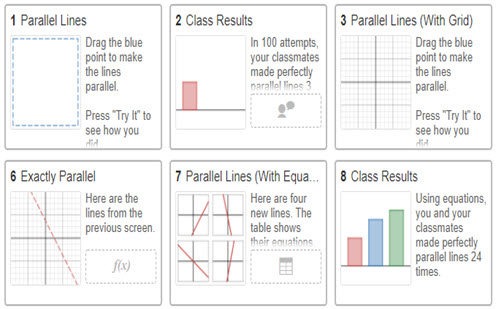Go to website

# Parallel lines and related angles

This GeoGebra applet interactive allows students to move the lines and observe the changes to the angles in and around the parallel lines. The task reinforces mathematical vocabulary and asks students to draw conclusions about the intersection of the transversal and parallel lines.

Year level(s) Year 7
Audience Teacher
Purpose Teaching resource
Format Interactive
Teaching strategies and pedagogical approaches Feedback, Mathematics investigation
Keywords parallel lines, transversal, alternate angles, corresponding angles

## Curriculum alignment

Curriculum connections Numeracy
Strand and focus Space
Topics Angles and geometric reasoning
AC: Mathematics (V9.0) content descriptions
AC9M7M04
Identify corresponding, alternate and co-interior relationships between angles formed when parallel lines are crossed by a transversal; use them to solve problems and explain reasons

AC9M7M05

Demonstrate that the interior angle sum of a triangle in the plane is 180° and apply this to determine the interior angle sum of other shapes and the size of unknown angles

Numeracy progression Understanding geometric properties (P6)